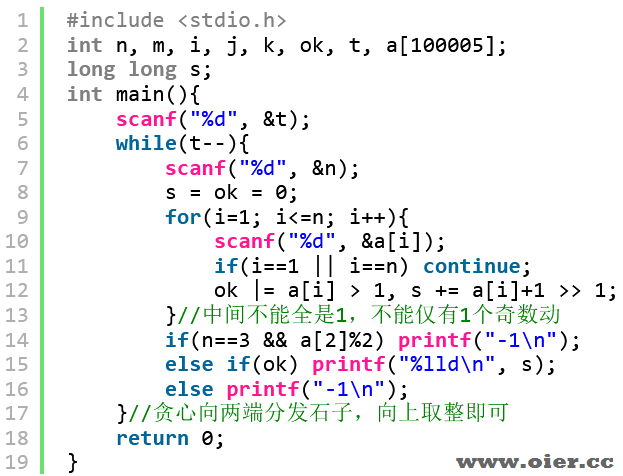CF1637C Andrew and Stones
181+

## 题意翻译

– $t$ 组数据，$1\leqslant t\leqslant 10^4$。
– $3\leqslant n,\sum n\leqslant 10^5$。
– $1\leqslant a_i\leqslant 10^9$。

Translated by Eason_AC

## 题目描述

Andrew has $n$ piles with stones. The $i$ -th pile contains $a_i$ stones. He wants to make his table clean so he decided to put every stone either to the $1$ -st or the $n$ -th pile.

Andrew can perform the following operation any number of times: choose $3$ indices $1 \le i < j < k \le n$ , such that the $j$ -th pile contains at least $2$ stones, then he takes $2$ stones from the pile $j$ and puts one stone into pile $i$ and one stone into pile $k$ .

Tell Andrew what is the minimum number of operations needed to move all the stones to piles $1$ and $n$ , or determine if it’s impossible.

## 输入输出格式

### 输入格式

The input contains several test cases. The first line contains one integer $t$ ( $1 \leq t \leq 10\,000$ ) — the number of test cases.

The first line for each test case contains one integer $n$ ( $3 \leq n \leq 10^5$ ) — the length of the array.

The second line contains a sequence of integers $a_1, a_2, \ldots, a_n$ ( $1 \leq a_i \leq 10^9$ ) — the array elements.

It is guaranteed that the sum of the values $n$ over all test cases does not exceed $10^5$ .

### 输出格式

For each test case print the minimum number of operations needed to move stones to piles $1$ and $n$ , or print $-1$ if it’s impossible.

## 输入输出样例

### 输入样例 #1

4
5
1 2 2 3 6
3
1 3 1
3
1 2 1
4
3 1 1 2

### 输出样例 #1

4
-1
1
-1

## 说明

In the first test case, it is optimal to do the following:

1. Select $(i, j, k) = (1, 2, 5)$ . The array becomes equal to $[2, 0, 2, 3, 7]$ .
2. Select $(i, j, k) = (1, 3, 4)$ . The array becomes equal to $[3, 0, 0, 4, 7]$ .
3. Twice select $(i, j, k) = (1, 4, 5)$ . The array becomes equal to $[5, 0, 0, 0, 9]$ . This array satisfy the statement, because every stone is moved to piles $1$ and $5$ .

There are $4$ operations in total.In the second test case, it’s impossible to put all stones into piles with numbers $1$ and $3$ :

1. At the beginning there’s only one possible operation with $(i, j, k) = (1, 2, 3)$ . The array becomes equal to $[2, 1, 2]$ .
2. Now there is no possible operation and the array doesn’t satisfy the statement, so the answer is $-1$ .

In the third test case, it’s optimal to do the following:

1. Select $(i, j, k) = (1, 2, 3)$ . The array becomes equal to $[2, 0, 2]$ . This array satisfies the statement, because every stone is moved to piles $1$ and $3$ .

The is $1$ operation in total.In the fourth test case, it’s impossible to do any operation, and the array doesn’t satisfy the statement, so the answer is $-1$ .

## 程序实现